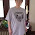## Thursday, April 5, 2018

### Kurt Cobain died on 4/5/1994

Kurt Donald Cobain (February 20, 1967 – April 5, 1994)

2+20+19+67=108
"Kurt Cobain" = 108 (Jewish Ordinal)
2+20+1+9+6+7=45
2+2+0+1+9+6+7=27

Died age 27 same as birth 2+2+0+1+9+6+7=27

4+5+19+94=122
4+5+1+9+9+4=32

Died on 4/5 same as birth 2+20+1+9+6+7=45

"Kurt Cobain" = 42 (Full Reduction)
"Kurt Cobain" = 33 (Chaldean)

Kurt Donald Cobain" = 56 (Jewish Reduction)
"Kurt Donald Cobain" = 614 (Jewish)
"Kurt Donald Cobain" = 216 (KFW Kabbalah)

April 5 is the 95th day of the year in the Gregorian calendar. There are 270 days remaining until the end of the year.

1.Nice Work Joan! Kurt Donald Cobain (Donald/Donald Trump) sums to "614" in Jewish (614, 6/14). June 14th is Trump's birthday.

"Kurt D Cobain"=71(Reverse Full Red.)Trump is "71" years old
How does Cobain's alleged death over 24 years ago have a parallel with Donald Trump???
"Twenty Four Years Ago"=888-(Sumerian)8x8x8=512
"Donald J Trump"=888(Sumerian)
"Twenty Four Years Ago"=512(Franc Baconis)
"Kurt Donald Cobain"=212(LCH Kabbalah)
"212" is the Area Code in Manhattan/New York City.

1.Oh wow! Thanks Marian! Did you see my work on Art Bell yet?
He died on Friday the thirteenth and his name adds up to 223.